### Home > MC1 > Chapter 6 > Lesson 6.3.2 > Problem6-134

6-134.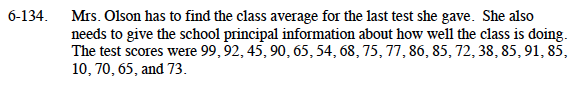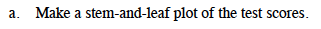Refer to the Math Notes box below for a reminder of how to create a stem-and-leaf plot.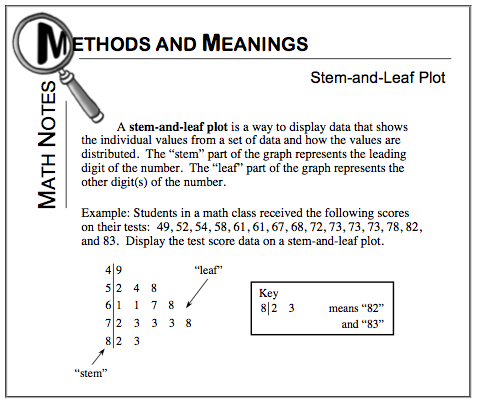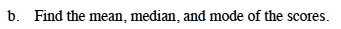Remember that the mean, or average, is calculated by adding all values and dividing by the number of values. The median is the middle number, and the mode is the most frequently repeated number of the data set.

The mean is 71.25, the median is 74, and the mode is 85.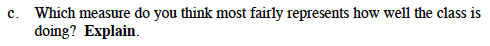Be sure to explain your answer and write down what you think!